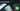# Hackerrank Solve Me First Solution

August 22nd, 2018
|

Solution for hackerrank warmup question solve me first in golang. In this blog I'll discuss my solution, what mistakes I initially made and what concepts I learned.

# Problem Statement

The question can be found at this link. The question is pretty straight forward. We have to take two integers as input, and print their sum. Couple of things to note here is

• The inputs are separated by new line character
• we are solving this in golang (assuming we have no or very little knowledge of golang)

# Challenges

• At first glance the platform opens up and we choose our preferred language as golang. The moment we do that, we get some 10-15 lines of code which are very unfamiliar to someone who is new to language.
• To solve this problem only thing we need to know is how to take input, show output, and do a sum.

# Solution

This is the template you get on hackerrank for this problem statement.

``````package main
import "fmt"

func solveMeFirst(a uint32,b uint32) uint32{
// Hint: Type return (a+b) below
}

func main() {
var a, b, res uint32
fmt.Scanf("%v\n%v", &a,&b)
res = solveMeFirst(a,b)
fmt.Println(res)
}``````
• First go ahead and delete everything in the pre-existing template.
• Now every Go code needs a package name, and if it is the main package it is supposed to named main (more on that in later blogs)
• we need to import the fmt library for standard input output operations.
• Next thing we need is our main function which automatically gets called and is necessary if your program is supposed to be run.
• we declare functions with func keyword, it takes no argument and has no return type

Up until now our code should look something like this

``````package main
import "fmt"

func main() {

}``````

Now it is time to write the program logic. First thing we need is declare three variables inside our main function. Two of them to hold the inputs and third for the sum. Add the declaration in side main function.

``var a,b,sum int;``

This bit of weird syntax is declaring three variables of integer type. If you are coming from java or c background this might look a bit different, but you'll get the hang of it. The best part is many a times we don't need to declare variables with types in golang. We will see examples of that in later blogs, or refer to the video below for more detailed explanation. Next we will take in the inputs. For taking input we have two options. Either take formatted input using Scanf or simple input using Scan. I will show you both. Type the following lines of code below your variable declaration.

``````fmt.Scanf("%d\n%d",&a,&b)
//fmt.Scan(&a,&b)``````

According to the problem statement we will be given two integers seperated by a new line, and that is exactly what "%d\n%d" is specifying here. %d means we want to take a integer input \n is the new line character followed by another integer. What follows next is not very staright forward to understand at first. It is saving those integers to memony where a and b is pointing to. It is related to the concept of pointers, which we will deal with in much greater detail in future. Second line which is commented is another way of taking input which directly takes the input as integers. Next we need to calculate the sum and print out the result

``````sum = a+b
fmt.Println(sum)``````

I guess it is pretty straight forward. We are taking the sum, and printing it out. We use Println to print out the result. Now your complete code should look like

``````import "fmt"

func main() {
var a,b,sum int;
fmt.Scanf("%d\n%d",&a,&b)
//fmt.Scan(&a,&b)
sum = a+b
fmt.Println(sum)
}``````